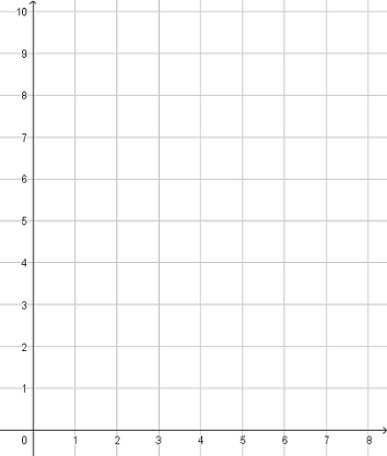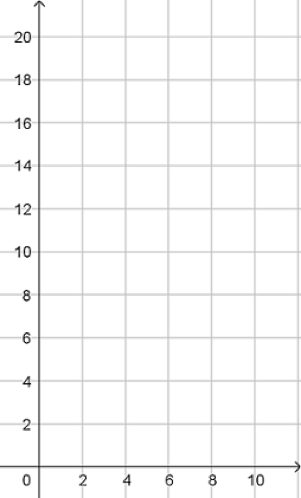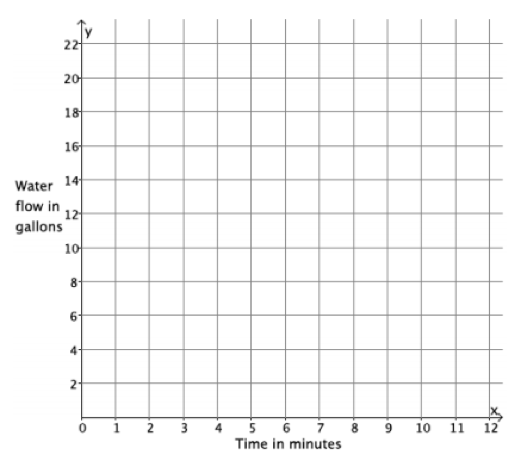# Functions

## Objective

Define and graph linear and nonlinear functions.

## Common Core Standards

### Core Standards

?

• 8.F.A.3 — Interpret the equation y = mx + b as defining a linear function, whose graph is a straight line; give examples of functions that are not linear. For example, the function A = s² giving the area of a square as a function of its side length is not linear because its graph contains the points (1,1), (2,4) and (3,9), which are not on a straight line.

?

• 7.EE.B.4

• 7.RP.A.2

## Criteria for Success

?

1. Graph a linear function using a table of values and coordinate points.
2. Graph a nonlinear function using a table of values and coordinate points.
3. Understand that a linear function consists of ordered pairs that, when graphed, lie on a straight line; points on a nonlinear function do not lie on a straight line.

## Tips for Teachers

?

Lessons 7 and 8 introduce students to linear vs. nonlinear functions. In Lesson 7, students see what examples of linear and nonlinear functions look like as graphs, and in Lesson 8, students will look at examples of functions in all representations and determine if they are linear or nonlinear.

#### Fishtank Plus

• Problem Set
• Student Handout Editor
• Vocabulary Package

## Anchor Problems

?

### Problem 1

It costs ${4}$ to enter a carnival and an additional ${2}$ for each activity you do once inside the carnival. The equation below represents the total cost spent at the carnival, $y$, as a function of the number of activities done, $x$.

$y=2x+4$

1. Create a table of values that represents some inputs and their corresponding outputs and use the table of values to sketch a graph of the function.1. Does this function appear to be a linear function?
2. What is the rate of change?
3. What is the initial value?

### Problem 2

The equation below represents the area of a square, $a$, as a function of its side length, $s$.

$a=s^2$

1. Create a table of values that represents some inputs and their corresponding outputs and use the table of values to sketch a graph of the function.1. Does this function appear to be a linear function?

## Problem Set

?The following resources include problems and activities aligned to the objective of the lesson that can be used to create your own problem set.

?

Water flows from a hose at a constant rate of 11 gallons every 4 minutes. The total amount of water that flows from the hose is a function of the number of minutes you are observing the hose.

1. Write an equation that describes the amount of water, ${y,}$ in gallons, that flows from the hose as a function of the number of minutes, ${x }$, you observe it.
2. Use the equation you wrote in part (a) to determine the amount of water that flows from the hose during an 8-minute period, a 4-minute period, and a 2-minute period.
3. The input of the function, $x$, is time in minutes, and the output of the function, $y$, is the amount of water that flows out of the hose in gallons. Write the inputs and outputs from part (b) as ordered pairs, and plot them as points on the coordinate plane.#### References

EngageNY Mathematics Grade 8 Mathematics > Module 5 > Topic A > Lesson 5Exit Ticket

Grade 8 Mathematics > Module 5 > Topic A > Lesson 5 of the New York State Common Core Mathematics Curriculum from EngageNY and Great Minds. © 2015 Great Minds. Licensed by EngageNY of the New York State Education Department under the CC BY-NC-SA 3.0 US license. Accessed Dec. 2, 2016, 5:15 p.m..

Modified by The Match Foundation, Inc.

?